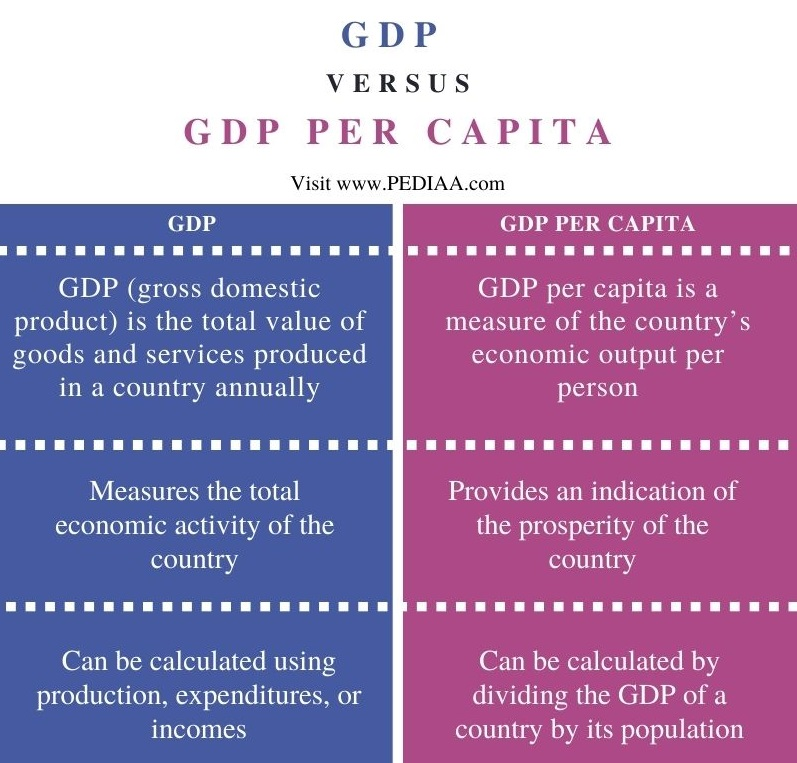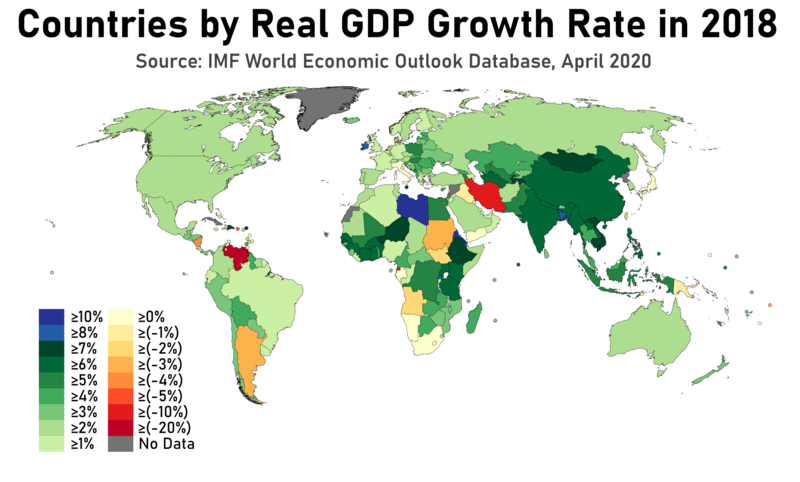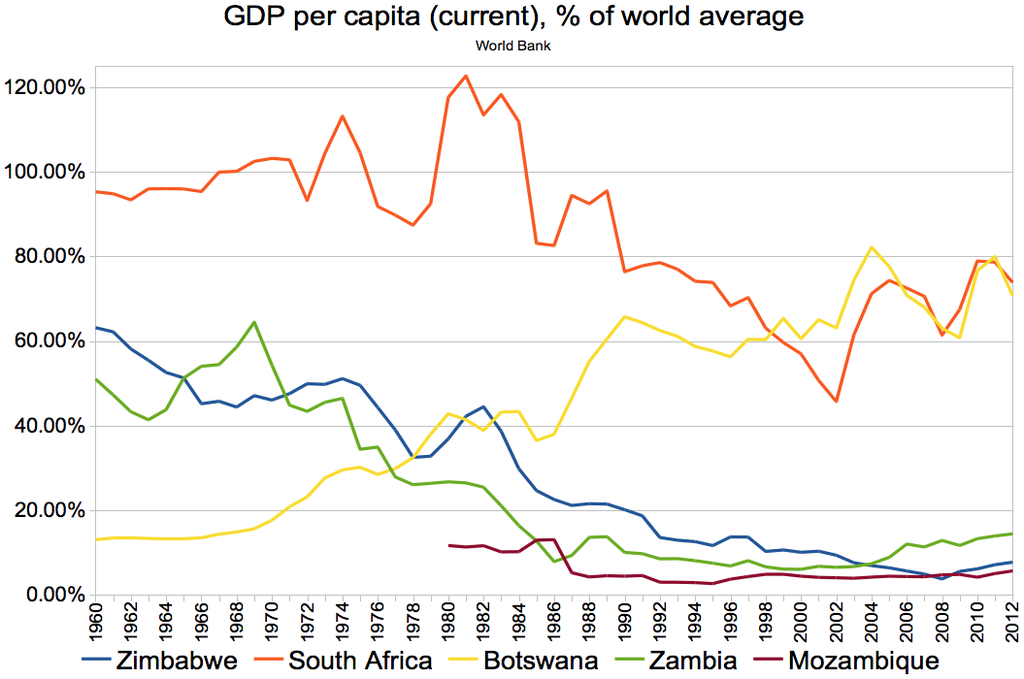# What is the Difference Between GDP and GDP Per Capita

The main difference between GDP and GDP per capita is that GDP is the total value of goods and services a country produces annually, whereas GDP per capita is a measure of the country’s economic output per person.

GDP and GDP per capita are two important measures the economists use to measure the size of a country’s economy and growth rate. While GDP measures the total economic activity of the country, GDP per capita provides an indication of the prosperity of the country.

### Key Areas Covered

1. What is GDP
– Definition, Features, Calculation
2. What is GDP per Capita
– Definition, Features
2. Relationship Between GDP and GDP Per Capita
– Outline of Common Features
3. Difference Between GDP and GDP Per Capita
– Comparison of Key Differences

### Key Terms

GDP, GDP Per Capita, Gross Domestic Product## What is GDP

GDP, which stands for Gross Domestic Product, is the total value of goods produced and services provided in a country during a specific period of time. GDP is generally calculated on a yearly basis, but sometimes it can be calculated on a quarterly basis as well.  Moreover, GDP gives a snapshot of the economy of the country and serves as a broad measure of overall domestic production. Economic experts use GDP to assess the size of an economy and its growth rate. GDP is an important measure for investors, businesses, and policy-makers.There are three methods to calculate GDP: using production, expenditures, or incomes. Using production is the most direct method as it sums the output of every enterprise to calculate the total. The expenditure method works on the principle that all products must be bought by someone, so the value of the total product should be equal to the total expenditure spent to buy things. Similarly, the income method works on the theory that the incomes of the producers must be equal to the value of their product, so the sum of all producers’ incomes should result in the GDP. Moreover, it’s also possible to adjust the GDP calculation to include inflation and population in order to learn deeper insights.

## What is GDP Per Capita

GDP Per Capita or Gross Domestic Product per capita is a measure of the country’s economic output per person. Moreover, GDP per Capita is calculated by dividing the GDP of a country by its population. We often use this measurement to assess the prosperity of a country and to analyze the standard of living. Economists often use GDP and GDP per capita to analyze the economic growth of a country.More developed industries and small, rich countries tend to have higher GDP per capita. Furthermore, a country with a high GPP but a larger population will naturally show a lower GDP per capita value. According to data from World Bank, Monaco, Liechtenstein, Luxembourg, Bermuda, Cayman Islands, and Switzerland are the six countries/territories with the highest GDP per capita.

## Relationship Between GDP and GDP Per Capita

• GDP is calculated by dividing the GDP of a country by its population.
• Moreover, a country with a higher GDP value may have a lower GDP per capita value if that country has a large population.

## Difference Between GDP and GDP Per Capita

### Definition

GDP is the total value of goods and services produced in a country annually, whereas GDP per capita is a measure of the country’s economic output per person.

### Use

GDP measures the total economic activity of the country, while GDP per capita provides an indication of the prosperity of the country.

### Calculation

There are three methods to calculate GDP: using production, expenditures, or incomes. In contrast, dividing the GDP of a country by its population results in GDP per capita value

### Conclusion

Both GDP and GDP per capita are two important economic measures. The main difference between GDP and GDP per capita is that GDP is the total value of goods and services a country produces annually whereas GDP per capita is a measure of the country’s economic output per person.

##### Reference:

1. Fernando, Jason. “Gross Domestic Product (Gdp).” Investopedia, 13 Sept. 2021.
2. “Gdp per Capita (Current Us\$).” Data – World Bank, 2021.

##### Image Courtesy:

1. “Countries by Real GDP Growth Rate in 2018” By Abdullah Ali Abbasi – Own work (CC BY-SA 4.0) via Commons Wikimedia
2. “GDP per capita (current), % of world average, 1960-2012; Zimbabwe, South Africa, Botswana, Zambia, Mozambique” By Underlying lk – Own work (CC BY-SA 3.0) via Commons Wikimedia